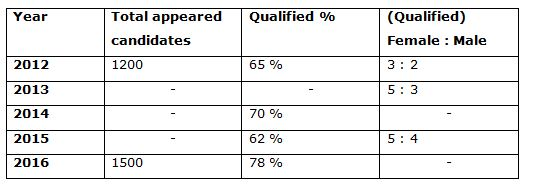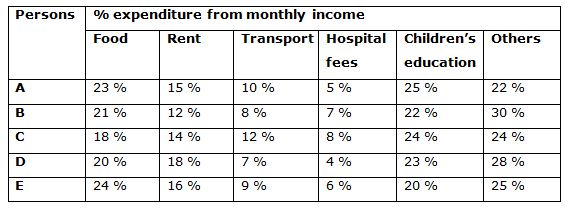# Quantitative Aptitude Questions (Data Interpretation) for SBI Clerk Mains 2018 Day-161

Dear Readers, SBI is conducting Online Examination for the recruitment of clerical cadre To enrich your preparation here we have providing new series of Data Interpretation – Quantitative Aptitude Questions. Candidates those who are appearing in SBI Clerk Mains Exams can practice these Quantitative Aptitude average questions daily and make your preparation effective.

[WpProQuiz 2977]

Click “Start Quiz” to attend these Questions and view Solutions

Directions (Q. 1 – 5): Study the following information carefully and answer the given questions: Following table shows the total number of candidates appeared for the examination over the years and the percentage of qualified candidates and the ratio of male and female qualified candidates among them.1. In 2016, the total number of female qualified candidates was 520. Total number of female qualified candidates is what percentage of total number of male qualified candidates in 2016?

a) 60 %

b) 80 %

c) 70 %

d) 65 %

e) 75 %

2. In 2017, the number of appeared candidates increased by 35 % while comparing with the previous year. If 68 % of appeared candidates in 2017 is qualified in the examination, then find the total number of candidates qualified in 2017?

a) 1453

b) 1421

c) 1345

d) 1377

e) None of these

3. In 2013, the respective ratio of number of appeared candidates to that of qualified candidates was 5:4. Total number of female qualified candidates is what percent of number of appeared candidates in the same year?

a) 50 %

b) 40 %

c) 65 %

d) 60 %

e) None of these

4. If the number of female qualified candidates in the year 2015 is 620, then find the total number of appeared candidates in the year 2015?

a) 1600

b) 1900

c) 1800

d) 1700

e) None of these

5. If the ratio of total number of candidates appeared for the examination in the year 2014 to 2016 is 4: 3 and the total number of male qualified candidates in the year 2013 is 360, then find the difference between the total number of qualified candidates in the year 2014 to that of total number of female qualified candidates in the year 2013?

a) 650

b) 800

c) 750

d) 900

e) None of these

Directions (Q. 6 – 10) Study the following information carefully and answer the given questions:

Following table shows the percentage expenditure (Food, Rent, Transport, Hospital fees, Children’s education and others) of 5 different persons.Income = Expenditure + Savings

6. If the monthly income of person A and C is Rs. 45000 and Rs. 60000 respectively and their percentage of savings are 20 % and 24 % respectively, then find the difference between the total expenditure spent on Food, Transport, Children’s education and Others of Person A to that of total expenditure spent by the person C except children’s education?

a) Rs. 6230

b) Rs. 7145

c) 5856

d) Rs. 6724

e) None of these

7. If the amount of money spent on Food and Transport together by B and D is Rs. 14500 and Rs. 17550 respectively and their saving are Rs. 10000, Rs. 15000 respectively, then find the ratio between the monthly income of B to that of D?

a) 3: 4

b) 4: 5

c) 2: 3

d) 5: 6

e) None of these

8. The ratio of monthly income of B and E is 2: 3. The amount of money spent on Rent by the person B is Rs. 5400 and savings of B is Rs. 15000. Find the amount spent by E on Food and Children’s education together?

a) Rs. 42500

b) Rs. 48700

c) Rs. 33200

d) 39600

e) None of these

9. If the amount of money spent on Children’s education by A is Rs. 9000 and the percentage of savings is 20 %, then find the monthly income of A?

a) Rs. 36000

b) 45000

c) Rs. 42000

d) Rs. 38000

e) None of these

10. The amount of money saved by the person E is Rs. 22500. Find the amount of money spent by E on Transport and Hospital fees together, if the percentage of saving of E is 25 %?

a) Rs. 15800

b) Rs. 16400

c) Rs. 14300

d) Rs. 10125

e) None of these

Direction (1-5)

Total number of female qualified candidates in 2016 = 520

Total qualified candidates in 2016 = 1500*(78/100) = 1170

Total number of male qualified candidates in 2016 = 1170 – 520 = 650

Required % = (520/650)*100 = 80 %

Total number of appeared candidates in the year 2017 = 1500*(135/100) = 2025

Total number of qualified candidates in the year 2017 = 2025*(68/100) = 1377

The ratio of number of appeared candidates to that of qualified candidates

= > 5: 4 = 5x and 4x

The ratio of female to male qualified candidates = 5: 3

Number of female qualified candidates = 4x * 5/8

Required percentage = (4x*5/8)/5x *100 = 50%

The number of female qualified candidates in the year 2015 = 620

The ratio of female to that of male qualified candidates in the year 2015 = 5: 4

5’s = 620

1’s = 124

Total number of qualified candidates in the year 2015 = 9’s = 1116

(62/100)*appeared candidates = 1116

Total number of appeared candidates in the year 2015 = 1116*(100/62) = 1800

The ratio of total number of candidates appeared for the examination in the year 2014 to 2016 = 4: 3

Total number of candidates appeared for the examination in the year 2016 = > 3’s = 1500

1’s = 500

Total number of candidates appeared for the examination in the year 2014

= > 4’s = 2000

Total number of candidates qualified for the examination in the year 2014

= > 2000*(70/100) = 1400

The total number of male qualified candidates in the year 2013 = 360

3’s = 360

1’s = 120

Total number of female qualified candidates in the year 2013 = 5’s = 600

Required difference = 1400 – 600 = 800

Direction (6-10)

The monthly income of person A = Rs. 45000

Total expenditure of person A = 45000*(80/100) = Rs. 36000

Total expenditure spent on Food, Transport, Children’s education and Others of Person A

=>36000* (23+10+25+22)/100

= > 36000*(80/100) = Rs. 28800

The monthly income of person C = Rs. 60000

Total expenditure of person C = 60000*(76/100) = Rs. 45600

Total expenditure spent by the person C except children’s education

= > 45600*(76/100) = Rs. 34656

Required difference = 34656 – 28800 = Rs. 5856

The amount of money spent on Food and Transport together by B = Rs. 14500

The savings of B = Rs. 10000

(29/100)*Expenditure of B = 14500

Expenditure of B = 14500*(100/29) = Rs. 50000

Monthly income of B = 50000 + 10000 = Rs. 60000

The amount of money spent on Food and Transport together by D = Rs. 17550

The savings of D = Rs. 15000

(27/100)*Expenditure of D = 17550

Expenditure of D = 17550*(100/27) = Rs. 65000

Monthly income of D = 65000 + 15000 = Rs. 80000

Required ratio = 60000: 80000 = 3: 4

The ratio of monthly income of B and E = 2: 3 (2x, 3x)

The amount of money spent on Rent by the person B = Rs. 5400

(12/100)*Expenditure of B = 5400

Expenditure of B = 5400*(100/12) = Rs. 45000

Savings of B = Rs. 15000

Monthly income of B = 45000 + 15000 = Rs. 60000

2x = 60000

x = 30000

Monthly income of E = 3x = Rs. 90000

The amount spent by E on Food and Children’s education together

= > 90000*(44/100) = Rs. 39600

The amount of money spent on Children’s education by A = Rs. 9000

(25/100)*Expenditure of A = 9000

Expenditure of A = 9000*(100/25) = Rs. 36000

% of savings = 20 %

(80/100)*Monthly income of A = 36000

Monthly income of A = 36000*(100/80) = Rs. 45000

The amount of money saved by the person E = Rs. 22500

Savings % of E = 25 %

(25/100)*Monthly income of E = 22500

Monthly income of E = 22500*(100/25) = Rs. 90000

The amount of money spent by E on Transport and Hospital fees together

= > 90000*(75/100) *(15/100) = Rs. 10125

Daily Practice Test Schedule | Good Luck

 Topic Daily Publishing Time Daily News Papers & Editorials 8.00 AM Current Affairs Quiz 9.00 AM Quantitative Aptitude “20-20” 11.00 AM Vocabulary (Based on The Hindu) 12.00 PM General Awareness “20-20” 1.00 PM English Language “20-20” 2.00 PM Reasoning Puzzles & Seating 4.00 PM Daily Current Affairs Updates 5.00 PM Data Interpretation / Application Sums (Topic Wise) 6.00 PM Reasoning Ability “20-20” 7.00 PM English Language (New Pattern Questions) 8.00 PM